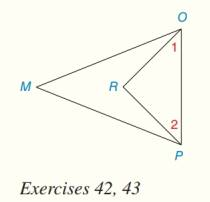Chapter 1.CR, Problem 42CR### Elementary Geometry for College St...

6th Edition
Daniel C. Alexander + 1 other
ISBN: 9781285195698

#### Solutions

Chapter
Section### Elementary Geometry for College St...

6th Edition
Daniel C. Alexander + 1 other
ISBN: 9781285195698
Textbook Problem
1 views

# Write two-column proofs for Review Exercises 39 to 46. Given: ∠ 1 is comp to ∠ M ∠ 2 is comp to ∠ M Prove: ∠ 1 ≅ ∠ 2To determine

To find:

The two-column proof for 12.

Explanation

Given:

The given figure is,

Figure (1)

1 is complementary to M and 2 is complementary to M.

Definition:

If the sum of two angles is 90° then the angles are known as complementary angles.

Approach:

The reasons for given statements is explained in table below.

 Statements Reasons 1. ∠1 is complementary to ∠M 1. Given 2. ∠2 is complementary to ∠M 2

### Still sussing out bartleby?

Check out a sample textbook solution.

See a sample solution

#### The Solution to Your Study Problems

Bartleby provides explanations to thousands of textbook problems written by our experts, many with advanced degrees!

Get Started